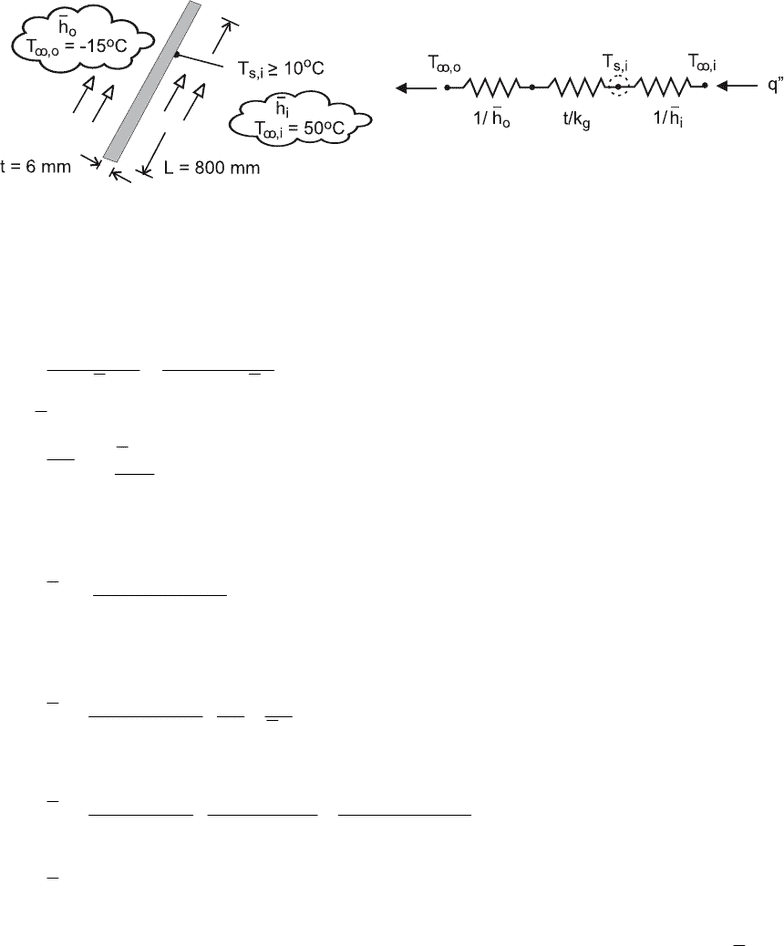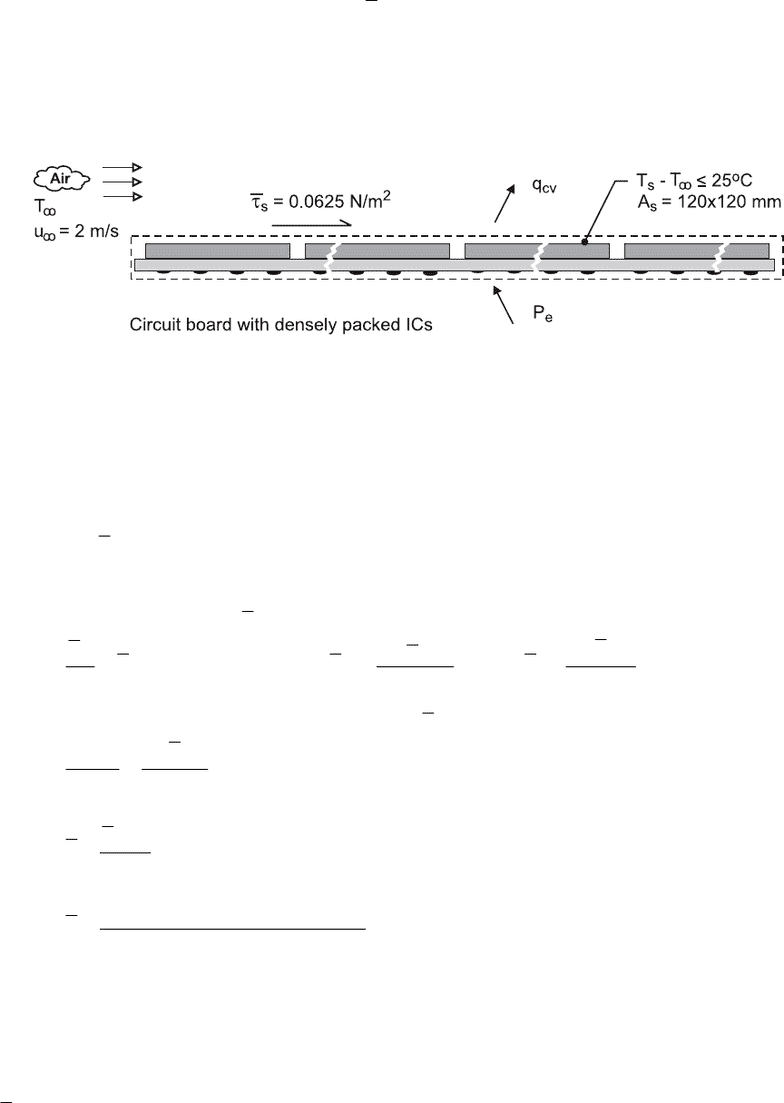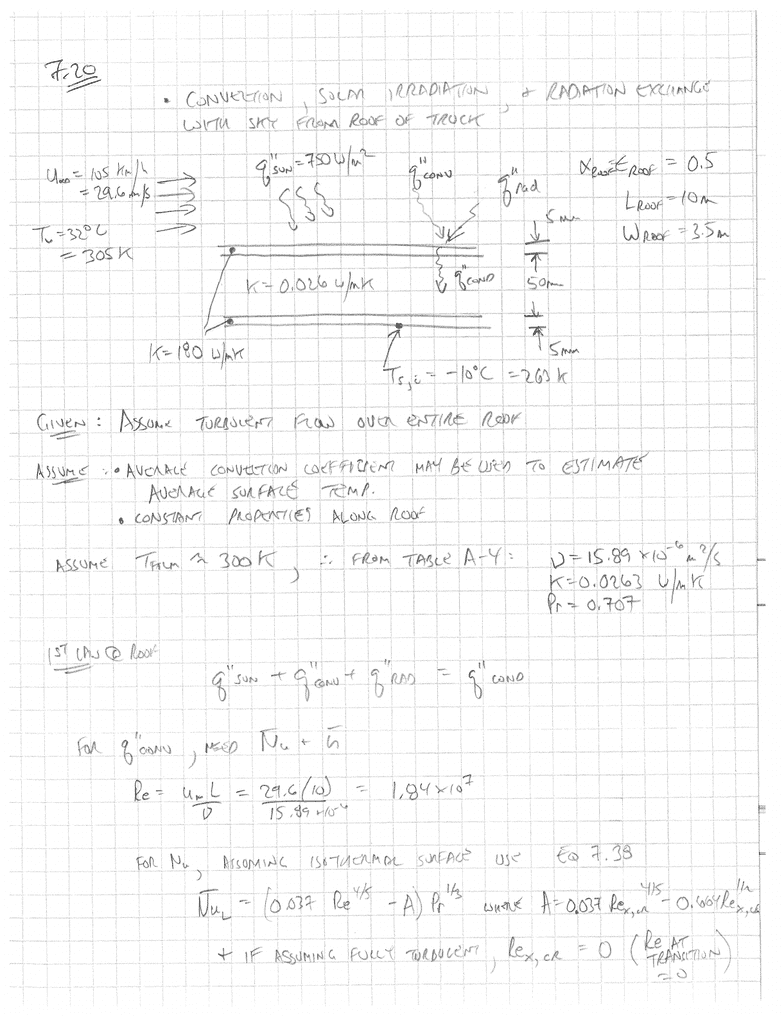Class Notes (1,100,000)
CA (650,000)
Carleton (20,000)
MECH (30)
wing (4)
Lecture 9

# MECH 4003 Lecture Notes - Lecture 9: Reynolds Number, Junction Temperature, Thermocouple

Department
Mechanical Engineering
Course Code
MECH 4003
Professor
wing
Lecture
9

This preview shows pages 1-3. to view the full 9 pages of the document.PROBLEM 6.43
KNOWN: Ambient, interior and dewpoint temperatures. Vehicle speed and dimensions of
windshield. Heat transfer correlation for external flow.
FIND: Minimum value of convection coefficient needed to prevent condensation on interior surface
of windshield.
SCHEMATIC:
ASSUMPTIONS: (1) Steady-state, (2) One-dimensional heat transfer, (3) Constant properties.
PROPERTIES: Table A-3, glass: kg = 1.4 W/mK. Prescribed, air: k = 0.023 W/mK, ν = 12.5 ×
10-6 m2/s, Pr = 0.70.
ANALYSIS: From the prescribed thermal circuit, conservation of energy yields
,i s,i s,i ,o
igo
TT TT
1/h t/k 1/h
∞∞
−−
=+
where o
h may be obtained from the correlation
L0.8 1/3
oL
hL
Nu 0.030Re Pr
k
==
With V = (70 mph × 1585 m/mile)/3600 s/h = 30.8 m/s, ReD = (30.8 m/s × 0.800 m)/12.5 × 10-6 m2/s
= 1.97 × 106 and
(
)
()
0.8 1/3
62
o0.023W / m K
h 0.030 1.97 10 0.70 83.1W / m K
0.800 m
=
From the energy balance, with Ts,i = Tdp = 10°C
()
()
1
s,i ,o
igo
,i s,i
TT t1
hkh
TT
⎛⎞
=+
⎜⎟
⎜⎟
⎝⎠
()
()
1
i2
10 15 C 0.006 m 1
h50 10 C 1.4W / m K 83.1W / m K
⎛⎞
⎜⎟=+
⎜⎟
−° ⋅
⎝⎠
2
i
h 38.3W / m K=⋅ <
COMMENTS: The output of the fan in the automobile’s heater/defroster system must maintain a
velocity for flow over the inner surface that is large enough to provide the foregoing value of i
h. In
addition, the output of the heater must be sufficient to maintain the prescribed value of T,i at this
velocity.

Only pages 1-3 are available for preview. Some parts have been intentionally blurred.PROBLEM 6.51
KNOWN: Average frictional shear stress of s
τ
=
0.0625 N/m2 on upper surface of circuit board with
densely packed integrated circuits (ICs)
FIND: Allowable power dissipation from the upper surface of the board if the average surface
temperature of the ICs must not exceed a rise of 25°C above ambient air temperature.
SCHEMATIC:
ASSUMPTIONS: (1) Steady-state conditions, (2) The modified Reynolds analogy is applicable, (3)
Negligible heat transfer from bottom side of the circuit board, and (4) Thermophysical properties
required for the analysis evaluated at 300 K,
PROPERTIES: Table A-4, Air (Tf = 300 K, 1 atm): ρ = 1.161 kg/m3, cp = 1007 J/kgK, Pr = 0.707.
ANALYSIS: The power dissipation from the circuit board can be calculated from the convection rate
equation assuming an excess temperature (Ts - T) = 25°C.
()
ss
qhAT T
=− (1)
The average convection coefficient can be estimated from the Reynolds analogy and the measured
average frictional shear stress s.
τ
2/3 s
ff2p
Ch
St Pr C St
2Vc
V/2
τ
ρ
ρ
=== (2,3,4)
With V = u and substituting numerical values, find h.
2/3
s2p
hPr
Vc
V
τ
ρ
ρ
=
sp 2/3
c
hPr
V
τ
=
()
22/3 2
0.0625 N / m 1007 J / kg K
h 0.707 39.7 W / m K
2m/s
×⋅
==
Substituting this result into Eq. (1), the allowable power dissipation is
()
22
q 39.7 W / m K 0.120 0.120 m 25 K 14.3 W=⋅×××=
<
COMMENTS: For this analysis using the modified or Chilton-Colburn analogy, we found Cf =
0.0269 and St = 0.0170. Using the Reynolds analogy, the results are slightly different with
2
h31.5W/mK=⋅ and q = 11.3 W.

Only pages 1-3 are available for preview. Some parts have been intentionally blurred.